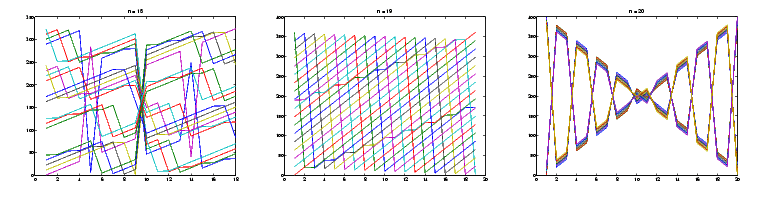MATLAB Function Referencemagic

Magic square

Syntax

• ```M` `=` `magic(n)
```

Description

```M = magic(n) ``` returns an `n`-by-`n` matrix constructed from the integers `1` through `n^2` with equal row and column sums. The order n must be a scalar greater than or equal to `3`.

Remarks

A magic square, scaled by its magic sum, is doubly stochastic.

Examples

The magic square of order 3 is

• ```M` `=` `magic(3)

M =

8    1    6
3    5    7
`    `4`    `9`    `2
```

This is called a magic square because the sum of the elements in each column is the same.

• ```` `sum(M)` `=

`     `15`    `15`    `15
```

And the sum of the elements in each row, obtained by transposing twice, is the same.

• ```` `sum(M')'` `=```
```
`     `15
`     `15
`     `15
```

This is also a special magic square because the diagonal elements have the same sum.

• ```sum(diag(M)) =

`     `15
```

The value of the characteristic sum for a magic square of order `n` is

• ```sum(1:n^2)/n
```

which, when `n = 3`, is `15`.

Algorithm

There are three different algorithms:

• `n` odd
• `n` even but not divisible by four
• `n` divisible by four

To make this apparent, type

• ```for` `n` `=` `3:20
`    `A` `=` `magic(n);
`    `r(n)` `=` `rank(A);
end
```

For `n` odd, the rank of the magic square is `n`. For `n` divisible by 4, the rank is `3`. For `n` even but not divisible by 4, the rank is `n/2 + 2`.

• ```[(3:20)',r(3:20)']
ans =
3     3
4     3
5     5
6     5
7     7
8     3
9     9
10     7
11    11
12     3
13    13
14     9
15    15
16     3
17    17
18    11
19    19
20     3
```

Plotting A for n = 18, 19, 20 shows the characteristic plot for each category.Limitations

If you supply `n` less than `3`, `magic` returns either a nonmagic square, or else the degenerate magic squares `1` and `[]`.

See Also

`ones`, `rand`

© 1994-2005 The MathWorks, Inc.